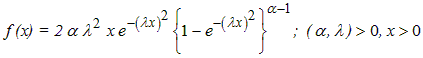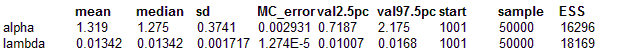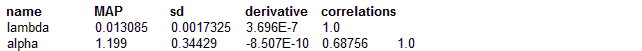#### BurrX modelmodel
{
for( i in 1 : N )
{
x[i] ~ dburrX(alpha, lambda)
}

# Prior distributions of the model parameters
# Uniform priors
alpha ~ dunif(0.1, 5.0)
lambda~ dunif(0.001, 1.0)
}

The data given here arose in tests on endurance of deep groove ball bearings.The data are the number of million revolutions before failure for each of the 23 ball bearings in the life test. The data are taken from Lawless (2003, pp. 99 ).

Lawless, J.F. (2003). Statistical Models and Methods for Lifetime data, Second edition, John Wiley & Sons, New York.

The MLE’s are using 'maxLik' package in R   alpha=1.1989515 lambda= 0.0130847

##### Data
``` list( N=23, x = c(17.88, 28.92, 33.00, 41.52, 42.12, 45.60, 48.80, 51.84, 51.96, 54.12, 55.56, 67.80, 68.64, 68.64, 68.88, 84.12, 93.12, 98.64, 105.12, 105.84, 127.92, 128.04, 173.40)) ```
##### Inits for chain 1
``` list(alpha=0.5, lambda=0.05) ```

##### Inits for chain 2
``` list(alpha=1.5, lambda=0.10) ```

ResultsMAP estimates are